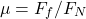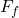# Coefficient of Friction

Tribology Wikipedia > Coefficient of Friction

Friction is a force resisting relative motion and may occur at the interface between the bodies, but may also occur within the bodies. The concept of friction coefficient was first formulated by Leonardo da Vinci and is defined according to the following equation:

(1)whereis the friction force andis the applied normal load.The magnitude of the coefficient of friction is determined by the properties of the surfaces, surroundings, surface features, presence of the lubricant, etc.

Generally, two kinds of friction coefficients are distinguished: static and dynamic. The static coefficient of friction is defined as the ratio of the maximum friction force prior to relative motion to the normal load. The dynamic (or kinetic) coefficient of friction is defined as the ratio of the friction force during sliding to the applied normal load. Frequently, the static friction coefficient is larger than the kinetic.

Friction also can be classified into dry and lubricated. Historically, lubrication was used to decrease overall friction coefficient by separating the surfaces by the lubricant layer. Since for regular engineering materials dry friction is significantly higher than the friction of the lubricant layers, the friction could be decreased by introducing a lubricant film. However, classical lubrication mechanism for friction reduction has reached its fundamental limit and currently solid lubricants get significant attention (see example) in attempts to reduce energy losses due to friction.

# Dry Coefficient of Friction

Guillaume Amontons (1663-1705) and Charles-Augustin Coulomb (1736-1806) formulated important laws of dry friction:

1. Friction force is proportional to the normal load (first Amonton’s law)
2. Friction force is independent of the apparent contact area (second Amonton’s law)
3. Kinetic friction is independent of sliding speed (Coulomb’s law)

According to the second Amonton’s law, the friction coefficient is a constant for a given pair of materials. These experimentally observed laws are considered to be fundamental, however recently it was shown that these laws are not always obeyed in various situations. This is especially true for nanoscale contacts, where all three laws can be broken. For example, friction of graphene may exhibit inverse friction force – normal load behavior breaking the first Amonton’s law. Second Amonton’s and Coulomb’s laws are not obeyed in Atomic Force Microscope (AFM) tip contacts. It was shown that in AFM tip contacts both the sliding speed and the apparent contact area influence the friction force.

Friction coefficients of various material combinations in atmosphere and vacuum are listed in Friction Coefficients table (the data was collected from various sources [1,2]).

# Lubricated Coefficient of Friction

Lubricated friction can be classified in three regimes: Boundary (BL), Mixed (ML) and Elastohydrodynamic (EHL). In contrast with dry friction, the lubricated friction depends on the operating conditions such as the sliding speed, lubricant viscosity, temperature, etc. The level of friction coefficient follows the following trend shown in Fig. 1.

A simple theory (load sharing concept) to calculate coefficient of friction in lubricated contacts was proposed by Johnson . This theory considers the total friction coefficient as a sum of two components, dry and lubricant friction weighted by the corresponding areas. The theory was shown to match experimental data quite well in some cases. For further details see .

To calculate friction coefficient in mixed lubrication regime, you can use the following matlab based codes: asperity based simulator, Tribology Simulator (standalone, no need in Matlab).

## Measuring Friction Coefficient

Tribometers are the devices which are typically used to measure friction. The first tribometer was probably invented by Leonardo da Vinci – the first tribologist. See a simple device developed from the grammofon on this video:

## References

. Mechanics and Physics of Precise Vacuum Mechanisms, E.A. Deulin, V.P. Mikhailov, Yu.V. Panfilov, R.A. Nevshupa.

. A simple theory of asperity contact in elastohydrodynamic lubrication. Wear. 1972;19:91-108, Johnson KL, Greenwood JA, Poon SY.

. On a Model for the Prediction of the Friction Coefficient in Mixed Lubrication Based on a Load-Sharing Concept with Measured Surface Roughness, Akchurin, A., Bosman, R., Lugt, P.M.

Save

Save

Save

Save

Save

Save

Save

Save

Save

Save

Save

•
•
•
•